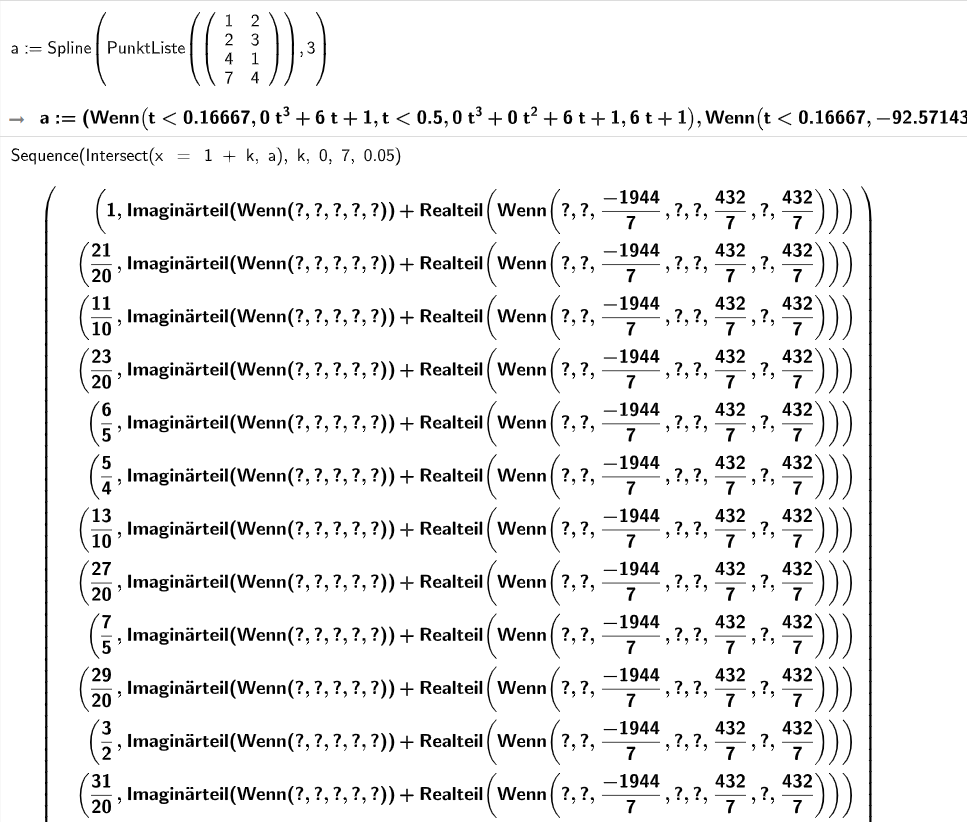# How to get an array of points from a curve?

Vladimir Mishakov shared this question 3 years ago

I am new to geogebra, so I may not understand some basics. I created a spline using "a=Spline(PointList({{1,2},{2,3},{4,1},{7,4}}),3)". Now I want to get the values of the points of this spline every 0.05 units in table format or something else. For example, like this:

(1, 2)

(1,05, 2,071375)

(1,1, 2,14242857142857)

...

What is the best way to do this?2

a way

``` l1=Sequence(Intersect(x = 1 + k, a), k, 0, 7, 0.05)
TableText(x(l1), y(l1), "v")```1